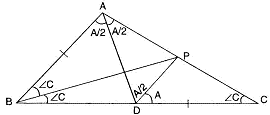# ABC is a triangle in which …## CBSE, JEE, NEET, NDA

Question Bank, Mock Tests, Exam Papers

NCERT Solutions, Sample Papers, Notes, Videos

ABC is a triangle in which angle B =angle 2C .d is a point on side BC such that ad bisects angle BAC and AB=CD. BE is per Pi sector of angle B find the measure of BAC

Sia 🤖 1 week, 3 days ago

In  {tex}\triangle{/tex}ABC,
BP bisects  {tex}\angle{/tex}ABC{tex}\therefore{/tex} {tex}\angle{/tex}ABP = {tex}\angle{/tex}PBC = {tex}\frac{1}{2}{/tex}{tex}\angle{/tex}B ={tex}\frac{1}{2}{/tex}(2{tex}\angle{/tex}C) = {tex}\angle{/tex}C . . . . (1)
In PBC,
{tex}\therefore{/tex} {tex}\angle{/tex}PBC = {tex}\angle{/tex}PCB (= ∠C)
{tex}\therefore{/tex} PB = PC . . . [Sides opposite to equal angles] . . .(2)
In {tex}\triangle{/tex}APB and {tex}\triangle{/tex}DPC,
AB = CD . . . [Given]
PB = PC . . .[From (2)]
{tex}\angle{/tex}ABP = {tex}\angle{/tex}DCP (= {tex}\angle{/tex}C)
{tex}\therefore{/tex} {tex}\triangle{/tex}APB = {tex}\triangle{/tex}DPC . . . . [By SAS property]
{tex}\therefore{/tex} {tex}\angle{/tex}BAP = CDP (= {tex}\angle{/tex}A) . . .[c.p.c.t.] . . . (3)
and AP = DP . . . [c.p.c.t.] . . . .(4)
In {tex}\triangle{/tex}APD,
As AP = DC . . . [From (4)]
{tex}\therefore{/tex} {tex}\angle{/tex}PDA = {tex}\angle{/tex}PAD = {tex}\frac{A}{2}{/tex}
{tex}\therefore{/tex} {tex}\angle{/tex}DPA =  {tex}\pi-\left(\frac{A}{2}+\frac{A}{2}\right)=\pi-A{/tex} . . . . (5)
From {tex}\angle{/tex}DPC
{tex}\angle{/tex}DPC = {tex}\pi{/tex} – (A + C)
{tex}\therefore{/tex} {tex}\angle{/tex}DPA = {tex}\pi{/tex} – {tex}\angle{/tex}DPC = {tex}\pi{/tex} – [{tex}\pi{/tex} – (A + C)] = A + C . . .(6)
From (5) and (6)
{tex}\pi{/tex} – A = A + C {tex}\therefore{/tex} 2A + C = {tex}\pi{/tex} . . . (7)
Again
A + B + C = {tex}\pi{/tex} . . .[Sum of three angles of a triangle = {tex}\pi{/tex}]
{tex}\therefore{/tex} A + 2C + C = {tex}\pi{/tex} . . .[As B = 2C]
{tex}\therefore{/tex} A + 3C = {tex}\pi{/tex} . . . (8)
Multiplying (7) by 3, we get
6A + 3C = 3{tex}\pi{/tex}
5A = 2{tex}\pi{/tex} . . .[By subtracting (8) from (9)]
{tex}\therefore{/tex}  {tex}A=\frac{2 \pi}{5}=\frac{2}{5} \times 180^{\circ}=72^{\circ}{/tex}
{tex}\therefore{/tex} {tex}\angle{/tex}BAC = 72o

Related Questions:## myCBSEguide

Trusted by 1 Crore+ Students

#### CBSE Test Generator

Create papers in minutes

Print with your name & Logo

3 Lakhs+ Questions

Solutions Included

Based on CBSE Blueprint

Best fit for Schools & Tutors

#### Work from Home

• Work from home with us
• Create questions or review them from home

No software required, no contract to sign. Simply apply as teacher, take eligibility test and start working with us. Required desktop or laptop with internet connection